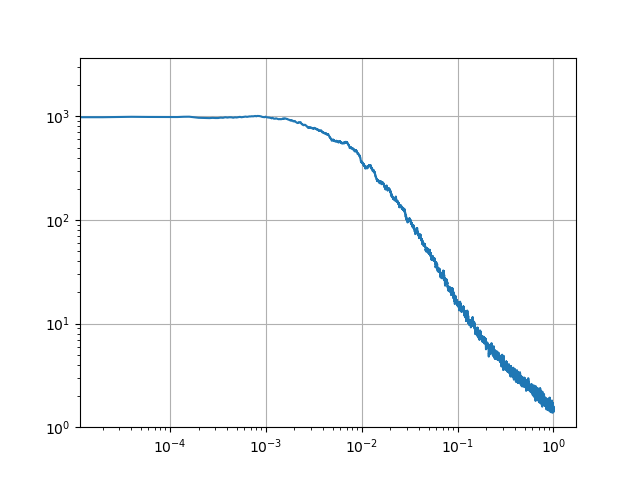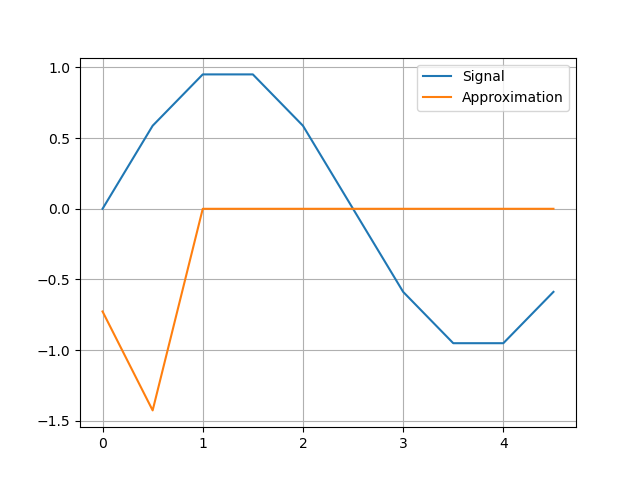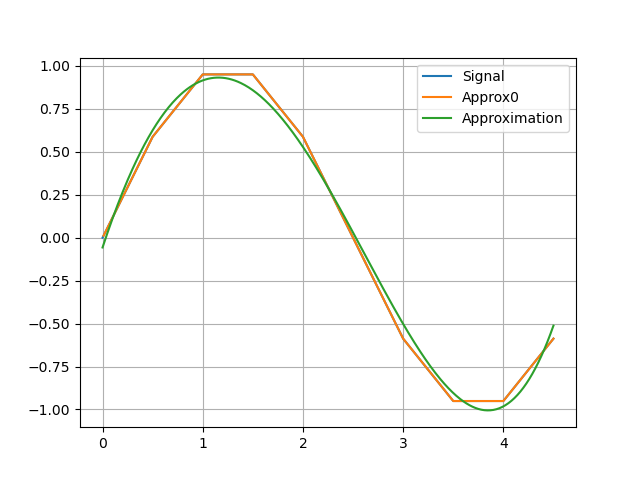# How to approximate a noisy spectrum

I have a noisy spectrum, and I want to get a -3db frequency and a -10db frequency somewhat accurately. It looks like this:How would you approach this task? Which approximation method and/or package would you use?

(Note: this is a log/log plot…)

1 Like

I’d fit this data to a chebyshev polynomial using Turing and ApproxFun.jl and then output the 3db and 10db points for each sample as generated quantities. After sampling you’ll have a Bayesian probability distribution over the quantities you want.

1 Like

The traditional DSP approach is to use a spectrum estimation technique such as Welch’s (implemented in DSP.jl as `welch_pgram`).

2 Likes

Thanks for pointing to ApproxFun.jl !

But I have only a vector of frequency, amplitude pairs, and not a function. ApproxFun needs a function as input. (I guess it must be differentiable, not so sure…).

How can I convert a vector of pairs to a function?

Using ApproxFun you can construct an approximator from coefficients. Those coefficients are your parameters. Then you eval the function at the frequency points and look at error between the ApproxFun and the measured data as your likelihood.

Take a look at the docs but it’s something like

``````Fun(Chebyshev(),mycoefs)
``````

Well, this doesn’t work:Code:

``````using PythonPlot, ApproxFun

f_ex = 0.2

N = 10
Ts = 0.5
tmax = (N-1) * Ts
t = 0:Ts:tmax
signal = sin.(2π * f_ex .* t) # sin (2π f t)

f = Fun(Chebyshev(), signal)

plot(t, signal, label = "Signal")
plot(t, f.(t), label = "Approximation")
legend()
grid(true)
``````

No, it’s interpreting your signal as coefficients. You need coefficients as unknown parameters and then find the ones which result in a value close to your data… Let me see if I can find an example I made…

I couldn’t find the example, but something like this:

``````
using Turing, ApproxFun

@model fitspectrum(freqs,responses)
mycoefs ~ MvNormal(zeros(12),10.0^2*I(12))
fitfun = Fun(Chebyshev(),mycoefs)
err ~ Gamma(3,0.1/2)
responses ~ MvNormal([fitfun(freqs[i]) for i in 1:length(freqs)],err^2*I(length(responses)))
# find the -3dB and -10dB points in the fitfun here
return(db3=db3val, db10=db10val)
end

model = fitspectrum(myfreqs,myresponses)

ss = sample(model,NUTS(600,.8),600)

mydbvals = generated_quantities(model,ss)

``````
1 Like

Note that there’s maybe a little more to it, for example I’m not sure but I think Fun(Chebyshev(),mycoefs) constructs a function that has a domain [-1,1] so you would probably want to transform your “freqs” to be in that range before passing them in, and then you’ll want to “untransform” the db3 and db10 values to get back to something physically meaningful.

Well, for now I will not use the Turing package, it is very large and I do not want to learn using more than one package at a time.

I managed to write some code that constructs a Chebychev polynom based on two vectors, x and y values of a function:

``````using PythonPlot, ApproxFun

f_ex = 0.2

N = 10
Ts = 0.5
tmax = (N-1) * Ts
t = 0:Ts:tmax
signal = sin.(2π * f_ex .* t) # sin (2π f t)

function discrete_signal(time)
if time < 0
return signal
elseif time > tmax
return signal[end]
end
for t0 in t
if time <= t0
return signal[1+Int64(t0/Ts)]
end
end
end

f = Fun(discrete_signal, Chebyshev(Interval(t1,t1[end])))

plot(t, signal, label = "Signal")
t1=0.0:0.01:4.5
plot(t1, discrete_signal.(t1), label = "Approx0")
plot(t1, f.(t1), label = "Approximation")
legend()
grid(true)
``````

But I did not find a way yet to limit the number of coefficients that are used. Any idea how to do that?

If you don’t want to use Turing, perhaps you would be ok with LsqFit and a point estimate. You will need something like that because you will have to describe which function you want. By default ApproxFun approximates to machine epsilon which is no different from just looking at your data.

Well, this code already does an approximation the way I want it, just by using the first 5 coefficients of the series:

``````using PythonPlot, ApproxFun

f_ex = 0.2

N = 10
Ts = 0.5
tmax = (N-1) * Ts
t = 0:Ts:tmax
signal = sin.(2π * f_ex .* t) # sin (2π f t)

function discrete_signal(time)
if time < 0
return signal
elseif time > tmax
return signal[end]
end
for t0 in t
if time > t0 && time <= t0+Ts
start = signal[1+Int64(t0/Ts)]
final = signal[2+Int64(t0/Ts)]
return start + (final-start)*(time-t0)/Ts
end
end
end

f = Fun(discrete_signal, Chebyshev(Interval(t,t[end])), 5)

plot(t, signal, label = "Signal")
t1=0.0:0.01:4.5
plot(t1, discrete_signal.(t1), label = "Approx0")
plot(t1, f.(t1), label = "Approximation")
legend()
grid(true)
``````But this is pretty complicated code …

So you just have the amplitude of the spectrum, right? No phase information? No time signal?

Well, I have time signal. I do not need the phase.

Can you use a subspace method such as N4SID? I think that is available in one of the packages of @baggepinnen .

Essentially, when you do not have a deterministic (control) input, you assume that the output is generated by a white noise, and N4SID will estimate the LTI system from the (unknown) noise to the output.

Agreed, what you need is a way to represent functions … and a way to find one that “fits through your data”. That’s two separate pieces of code in this case. ApproxFun.jl lets you represent generic functions in numerically stable ways. Turing lets you find ones that “fit the data”. If you don’t want to use Turing, you still need some way to describe “fitting the data”. Either use LsqFit or directly call something like Optim to optimize the “fit”.

ApproxFun by default will do a fit, but its sense of “fit” is “fits exactly through the data to within machine epsilon”. That’s not the kind of fit you’re looking for in this case.

For LsqFit you’d do something like:

``````function model(x,p)
f = Fun(Chebyshev(),p)
y = f.(x)
end

curve_fit(model,yourx,youry,p0)
``````

This is just not true. By using the first 5 coefficients I get a nice approximation already.

This is a nice property of Chebyshev polynomials, that they truncate well… but the computation that is being done by ApproxFun fits the data to machine epsilon, that’s why it chooses to use however many coefficients it chooses to use… and that’s the source of the inefficiency in some sense.

Well, just learned that with this notation you can avoid calculating more coefficients than you need:

``````f = Fun(discrete_signal, Chebyshev(Interval(t,t[end])), 5)
``````
1 Like

Nice! I wonder what calculation it’s doing there… maybe it is doing least squares internally for a fixed coefficient size, if so that’s perfect for what you need.

The next step is to use root finding to find what freq corresponds to response of -3dB and -10dB. Root finding can be done with Roots.jl